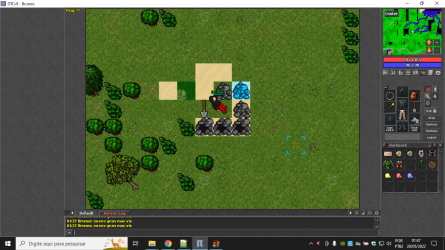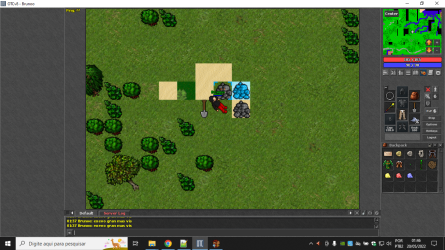# TFS 1.X+Script Shovel 1.5 7.72

#### bpm91

##### Well-Known Member
anyone help with shovel script? I would like it to work normally in the holes, but there are places in the desert that I need to open the sand, the problem is that now all the normal holes are turning into sand and then the area hole turns into sand again

Lua:
``````local holes = {468, 481, 483}
local sandHoles = {231} -- add sand ids
local sandAID = 2554
function onUse(player, item, fromPosition, target, toPosition, isHotkey)
local tile = Tile(toPosition)
if not tile then
return false
end

local ground = tile:getGround()
if not ground then
return false
end

local groundId = ground:getId()
if table.contains(holes, groundId) or (ground:getActionId() == sandAID and table.contains(sandHoles, groundId)) then
ground:transform(489)
ground:decay()

toPosition.z = toPosition.z + 1
tile:relocateTo(toPosition)
elseif groundId == 231 then
local randomValue = math.random(1, 100)
if randomValue == 1 then
Game.createItem(2159, 1, toPosition)
elseif randomValue > 95 then
Game.createMonster("Scarab", toPosition)
end
toPosition:sendMagicEffect(CONST_ME_POFF)
else
return false
end

return true
end``````Solution
try that

Lua:
``````local holes = {468, 481, 483} -- holes opened by shovel

function onUse(player, item, fromPosition, target, toPosition, isHotkey)
local tile = Tile(toPosition)
if not tile then
return false
end

local ground = tile:getGround()
if not ground then
return false
end

local groundId = ground:getId()
if table.contains(holes, groundId) then
ground:transform(groundId + 1)
ground:decay()

toPosition.z = toPosition.z + 1
tile:relocateTo(toPosition)
elseif groundId == 231 then
local randomValue = math.random(1, 100)
if target.actionid == 100 and randomValue <= 20 then
target:transform(489)
target:decay()...``````

#### mano368

##### Intermediate OT User
try that

Lua:
``````local holes = {468, 481, 483} -- holes opened by shovel

function onUse(player, item, fromPosition, target, toPosition, isHotkey)
local tile = Tile(toPosition)
if not tile then
return false
end

local ground = tile:getGround()
if not ground then
return false
end

local groundId = ground:getId()
if table.contains(holes, groundId) then
ground:transform(groundId + 1)
ground:decay()

toPosition.z = toPosition.z + 1
tile:relocateTo(toPosition)
elseif groundId == 231 then
local randomValue = math.random(1, 100)
if target.actionid == 100 and randomValue <= 20 then
target:transform(489)
target:decay()
elseif randomValue < 3 then
Game.createItem(2159, 1, toPosition)
elseif randomValue > 90 then
Game.createMonster("Scarab", toPosition)

end
toPosition:sendMagicEffect(CONST_ME_POFF)
else
return false
end

return true
end``````

#### bpm91

##### Well-Known Member
No work, i dont open hole in tombs ankrahmun :/

Last edited:

#### mano368

##### Intermediate OT User
No work, i dont open hole in tombs ankrahmun :/
If you are talking about transform sand in hole, you need to inset via mapeditor actionid 100 in the sand where you want

•bpm91

Workty

Replies
1
Views
358
T
Replies
14
Views
574
Tibia Demon
T
Replies
0
Views
184
Replies
3
Views
152
Replies
0
Views
186